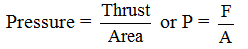# Physics

### Chapter : 2. Force & Laws Of Motion

#### Thrust And Pressure

(A) Thrust :
The force acting normally on surface is called 'thrust'.
This is a vector quantity.
It is measured in newton (N).
(B) Pressures :
The thrust on an unit area of a surface is called 'pressure'.Unit : The SI unit of pressure is newton per meter square or N/m2, other units of pressure are pascal and bar.
One Pascal : One pascal is defined as the pressure exerted on a surface area of 1m2 by a thrust of 1 newton.
i.e. 1 Pascal = 1 N/m2
Some examples based on pressure
Example. Inserting a pointed nail in a wooden block is an easier task than to insert a rod inside a wooden block with the same force because the nail has a smaller area and thus it will experience more pressure even with the same force.
Example. A sharp knife cuts better than a blunt knife.
Example. While walking, a man exerts more pressure on the ground in comparison to when he is standing.
Pressure in fluids
A substance that can flow is called a 'fluid'.
liquid and gases are considered as fluids